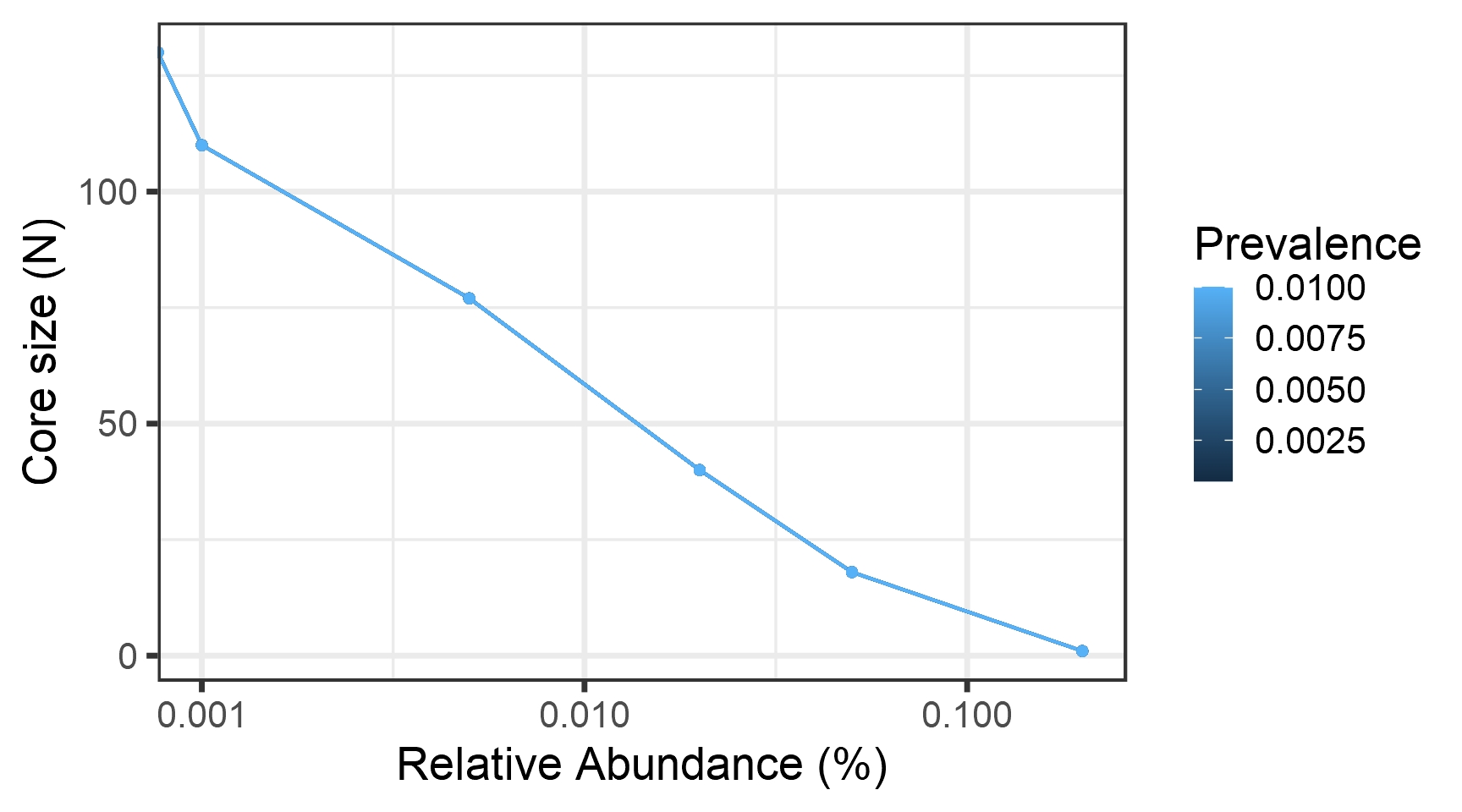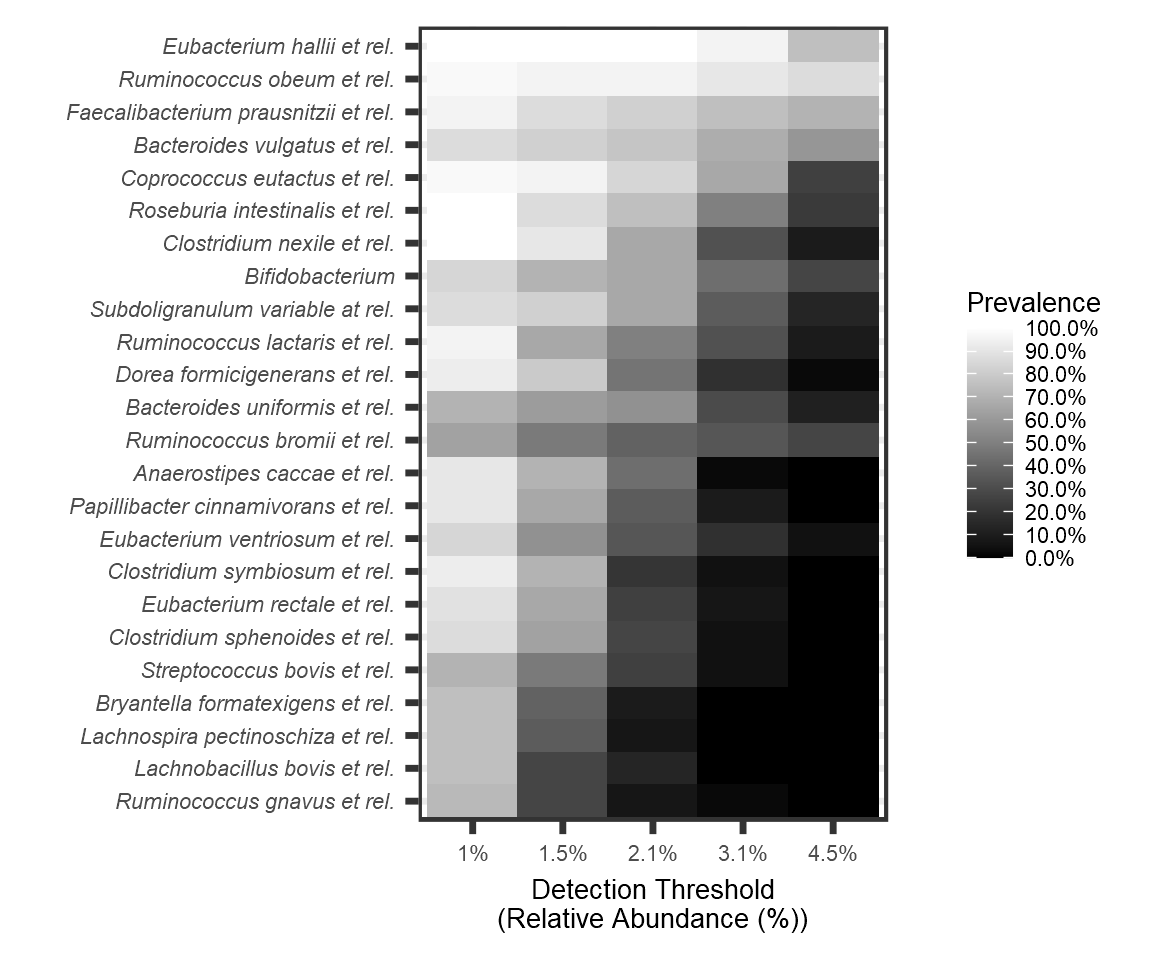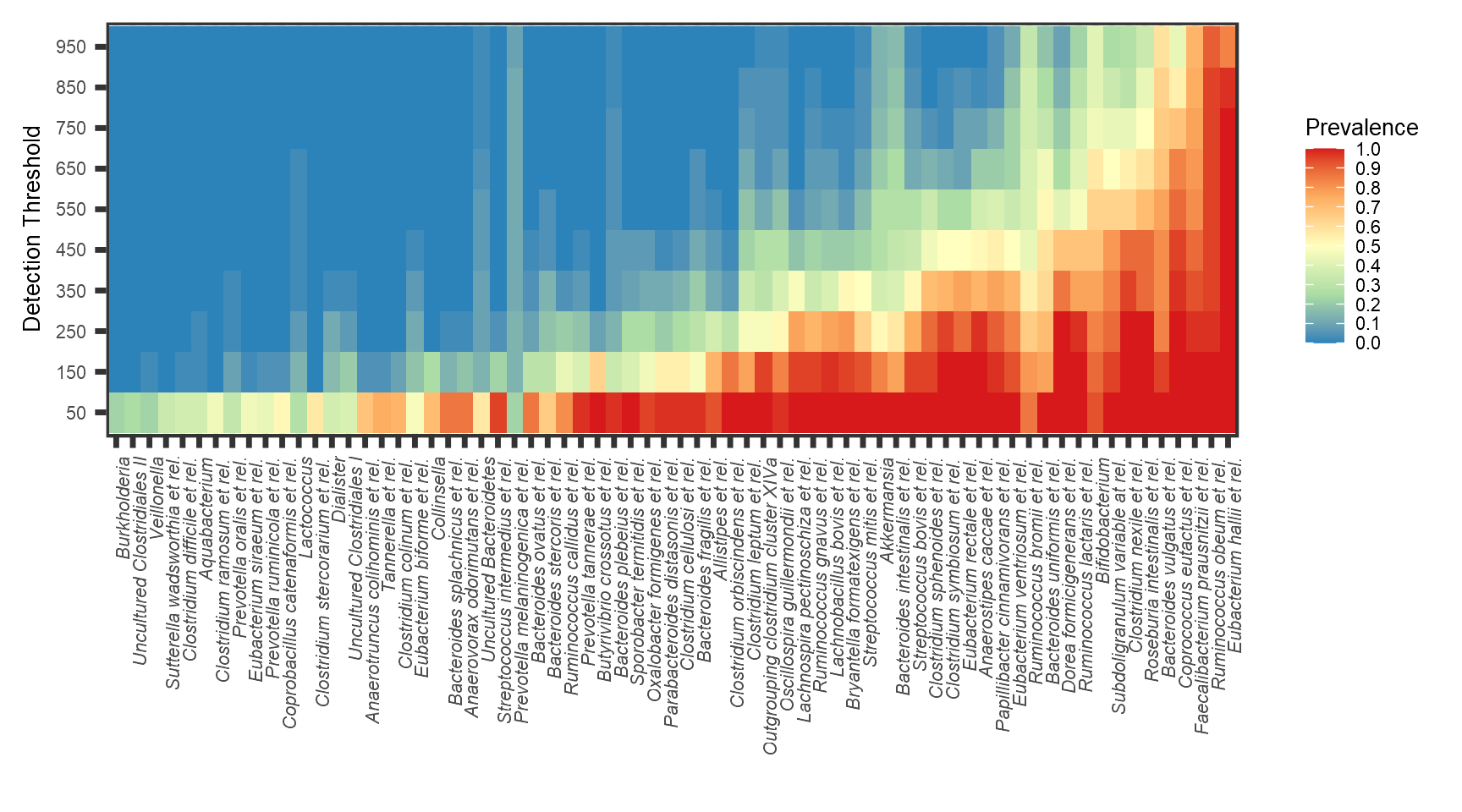See also related functions for the analysis of rare and variable taxa (rare_members; rare_abundance; rare_members; rare_abundance; low_abundance).

``````library("devtools")
#install_github("microbiome/microbiome")``````

# HITChip Data

``````# Load data
library(microbiome)
data(peerj32)

# Rename the data
pseq <- peerj32\$phyloseq

# Calculate compositional version of the data
# (relative abundances)
pseq.rel <- microbiome::transform(pseq, "compositional")``````

## Prevalence of taxonomic groups

Relative population frequencies; at 1% compositional abundance threshold:

``head(prevalence(pseq.rel, detection = 1/100, sort = TRUE))``
``````## Roseburia intestinalis et rel.     Eubacterium hallii et rel.     Clostridium nexile et rel.     Ruminococcus obeum et rel.
##                      1.0000000                      1.0000000                      1.0000000                      0.9772727
##   Coprococcus eutactus et rel.  Ruminococcus lactaris et rel.
##                      0.9772727                      0.9545455``````

Absolute population frequencies (sample count):

``head(prevalence(pseq.rel, detection = 1/100, sort = TRUE, count = TRUE))``
``````## Roseburia intestinalis et rel.     Eubacterium hallii et rel.     Clostridium nexile et rel.     Ruminococcus obeum et rel.
##                             44                             44                             44                             43
##   Coprococcus eutactus et rel.  Ruminococcus lactaris et rel.
##                             43                             42``````

## Core microbiota analysis

If you only need the names of the core taxa, do as follows. This returns the taxa that exceed the given prevalence and detection thresholds.

``core.taxa.standard <- core_members(pseq.rel, detection = 0, prevalence = 50/100)``

A full phyloseq object of the core microbiota is obtained as follows:

``pseq.core <- core(pseq.rel, detection = 0, prevalence = .5)``

We can also collapse the rare taxa into an “Other” category

``pseq.core2 <- aggregate_rare(pseq.rel, "Genus", detection = 0, prevalence = .5)``

Retrieving the core taxa names from the phyloseq object:

``core.taxa <- taxa(pseq.core)``

## Core abundance and diversity

Total core abundance in each sample (sum of abundances of the core members):

``core.abundance <- sample_sums(core(pseq.rel, detection = .01, prevalence = .95))``

## Core line plots

Determine core microbiota across various abundance/prevalence thresholds with the blanket analysis (Salonen et al. CMI, 2012) based on various signal and prevalences.

``````# With compositional (relative) abundances
det <- c(0, 0.1, 0.5, 2, 5, 20)/100
prevalences <- seq(.05, 1, .05)
#ggplot(d) + geom_point(aes(x, y)) + scale_x_continuous(trans="log10", limits=c(NA,1))

plot_core(pseq.rel,
prevalences = prevalences,
detections = det,
plot.type = "lineplot") +
xlab("Relative Abundance (%)")``````## Core heatmaps

This visualization method has been used for instance in Intestinal microbiome landscaping: Insight in community assemblage and implications for microbial modulation strategies. Shetty et al. FEMS Microbiology Reviews fuw045, 2017.

Note that you can order the taxa on the heatmap with the taxa.order argument.

``````# Core with compositionals:
library(RColorBrewer)
library(reshape)

prevalences <- seq(.05, 1, .05)

detections <- round(10^seq(log10(0.01), log10(.2), length = 9), 3)

# Also define gray color palette
gray <- gray(seq(0,1,length=5))

#Added pseq.rel, I thin... must be checked if it was in the the rednred version,; where it is initialized
#pseq.rel<- microbiome::transform(pseq, 'compositional')
#min-prevalence gets the 100th highest prevalence
p <- plot_core(pseq.rel,
plot.type = "heatmap",
colours = gray,
prevalences = prevalences,
detections = detections,
min.prevalence = prevalence(pseq.rel, sort = TRUE)) +
labs(x = "Detection Threshold\n(Relative Abundance (%))") +

#Adjusts axis text size and legend bar height
theme(axis.text.y= element_text(size=8, face="italic"),
axis.text.x.bottom=element_text(size=8),
axis.title = element_text(size=10),
legend.text = element_text(size=8),
legend.title = element_text(size=10))

print(p)````````````# Core with absolute counts and horizontal view:
# and minimum population prevalence (given as percentage)
detections <- seq(from = 50, to = round(max(abundances(pseq))/10, -1), by = 100)

p <- plot_core(pseq, plot.type = "heatmap",
prevalences = prevalences,
detections = detections,
colours = rev(brewer.pal(5, "Spectral")),
min.prevalence = .2, horizontal = TRUE) +
theme(axis.text.x= element_text(size=8, face="italic", hjust=1),
axis.text.y= element_text(size=8),
axis.title = element_text(size=10),
legend.text = element_text(size=8),
legend.title = element_text(size=10))

print(p)``````# Core Microbiota using Amplicon data

## Make phyloseq object

This tutorial is useful for analysis of output files from (Mothur), (QIIME or QIIME2) or any tool that gives a biom file as output. There is also a simple way to read comma seperated (*.csv) files.

Simple comma seperated files:

``````library(microbiome)

otu.file <-
system.file("extdata/qiita1629_otu_table.csv",
package='microbiome')

tax.file <- system.file("extdata/qiita1629_taxonomy_table.csv",
package='microbiome')

meta.file <- system.file("extdata/qiita1629_mapping_subset.csv",
package='microbiome')

otu.file=otu.file,
taxonomy.file=tax.file,

Biom file:

``````# Read the biom file
biom.file <-
system.file("extdata/qiita1629.biom",
package = "microbiome")

meta.file <-
system.file("extdata/qiita1629_mapping.csv",
package = "microbiome")
# Make phyloseq object
``````otu.file <- system.file(